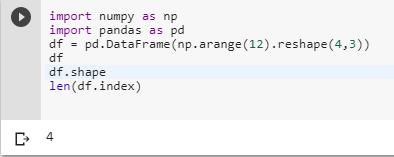2 views
in Python

I'm trying to get the number of rows of dataframe df with Pandas, and here is my code.

### Method 1:

total_rows = df.count

print total_rows +1

### Method 2:

total_rows = df['First_columnn_label'].count

print total_rows +1

Both the code snippets give me this error:

TypeError: unsupported operand type(s) for +: 'instancemethod' and 'int'

What am I doing wrong?

by (106k points)
• You should use .shape method to get the output or len(DataFrame.index). However len(DataFrame.index) will be the fastest way to get the answer.

import numpy as np

import pandas as pd

df = pd.DataFrame(np.arange(12).reshape(4,3))

df.shape

len(df.index)